# Grade 8 Math STAAR Review Activities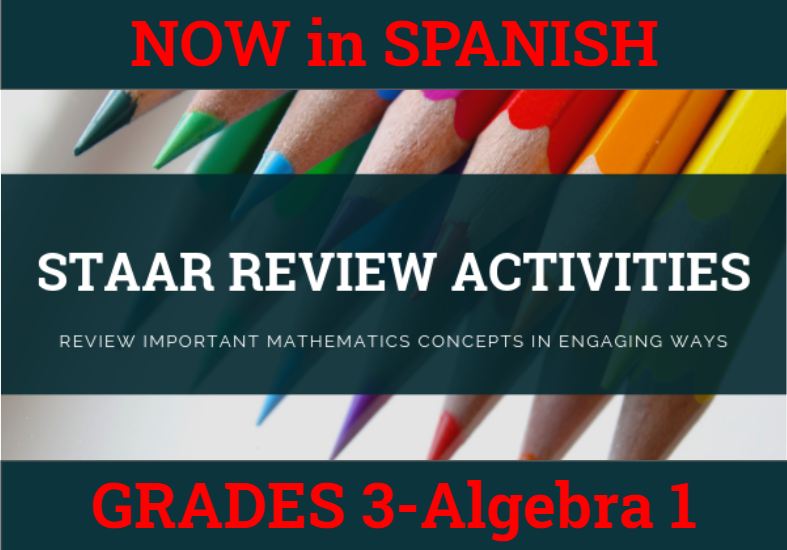How might we purposefully and effectively review important mathematics concepts for the Grade 8 Math STAAR assessment? The ESC-18 Math Team has created a variety of activities where students practice and apply important grade-level TEKS aligned topics to cement their learning.

### Please See Our Pricing and Instructions to Purchase our STAAR Activities

 8.2D Ordering Real Numbers Card Set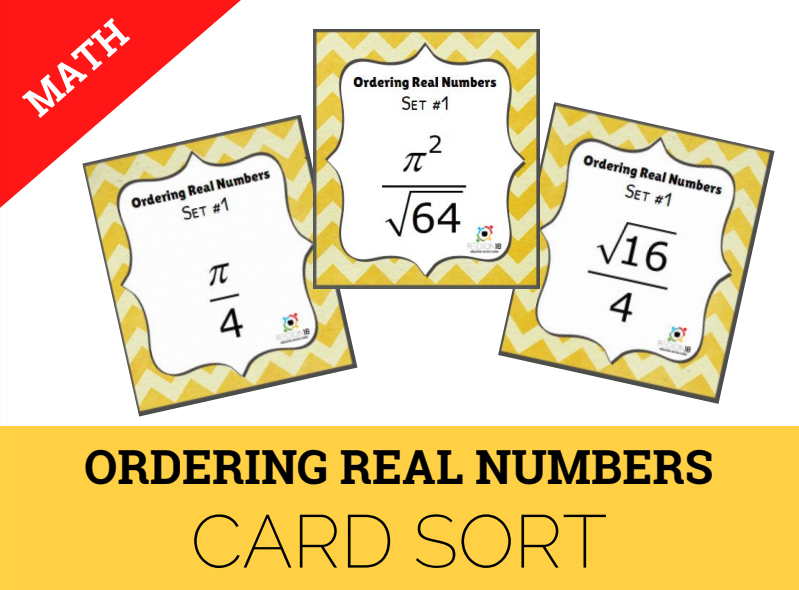Order a set of real numbers. 8.3C 8.10C Algebraic Representations for Transformations Question Stack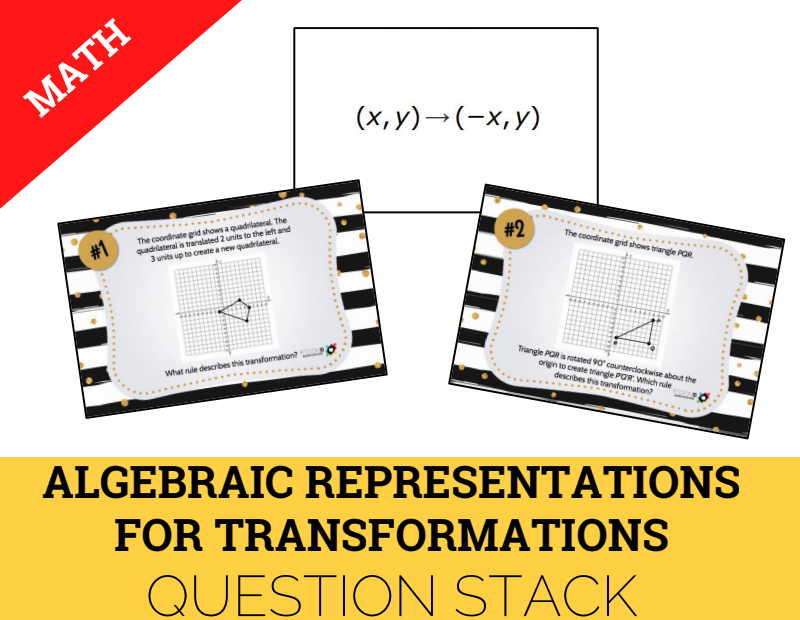Use an algebraic representation to explain the effects of dilations, translations, reflections, and rotations. 8.4B 8.4C 8.5D Analyzing Proportional and Non-Proportional Relationships True/False Sort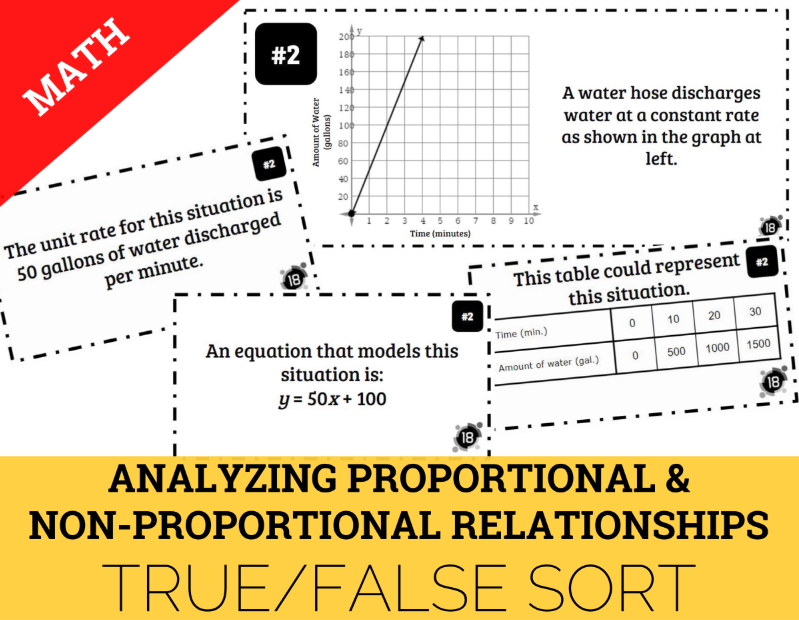Graph proportional and non-proportional relationships and interpret the rate of change for these situations. Use data from a table or graph to determine rate of change and y-intercept. Use a trend line to make predictions. 8.5G Function or Not Card Sort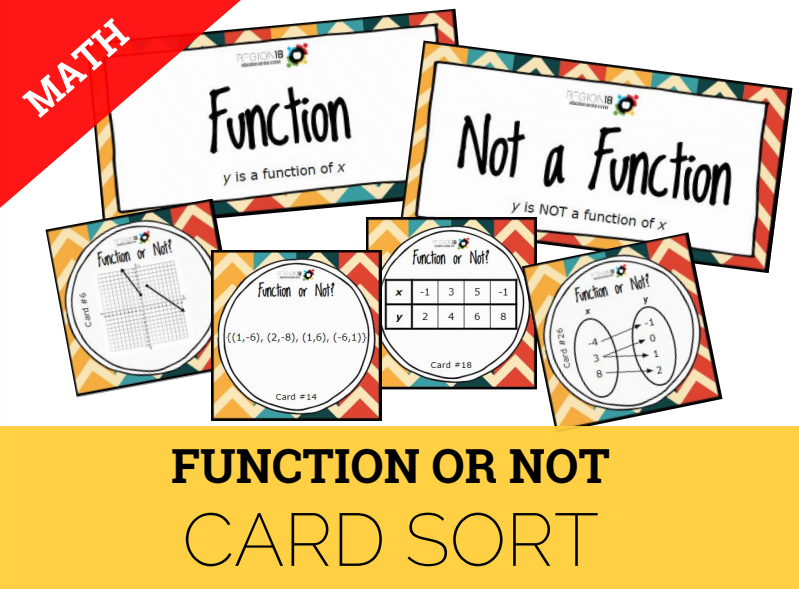Identify functions using sets of ordered pairs, tables, mappings, and graphs. 8.5I Linear Equations Scavenger Hunt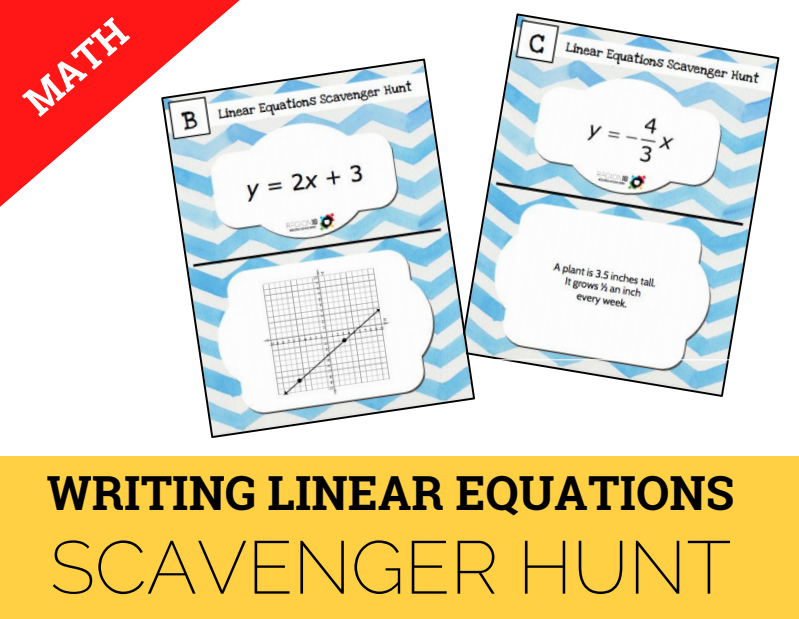Write an equation in the form y = mx + b to model a linear relationship between two quantities using verbal, numerical, tabular, and graphical representations. 8.7A Volume of Cylinders, Cones, & Spheres Sum It Up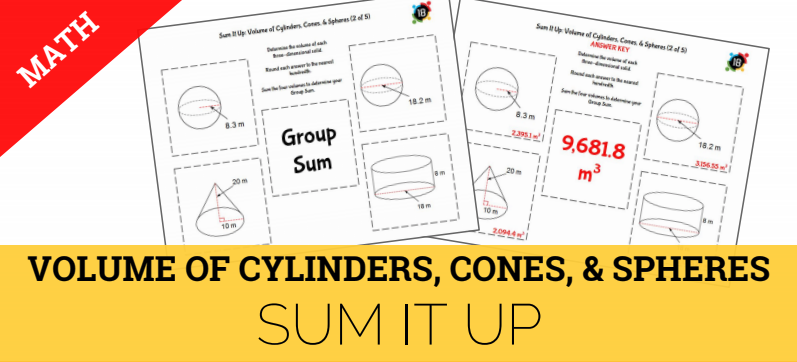Solve problems involving the volume of cylinders, cones, and spheres. 8.7B Surface Area of Prisms & Cylinders Dominoes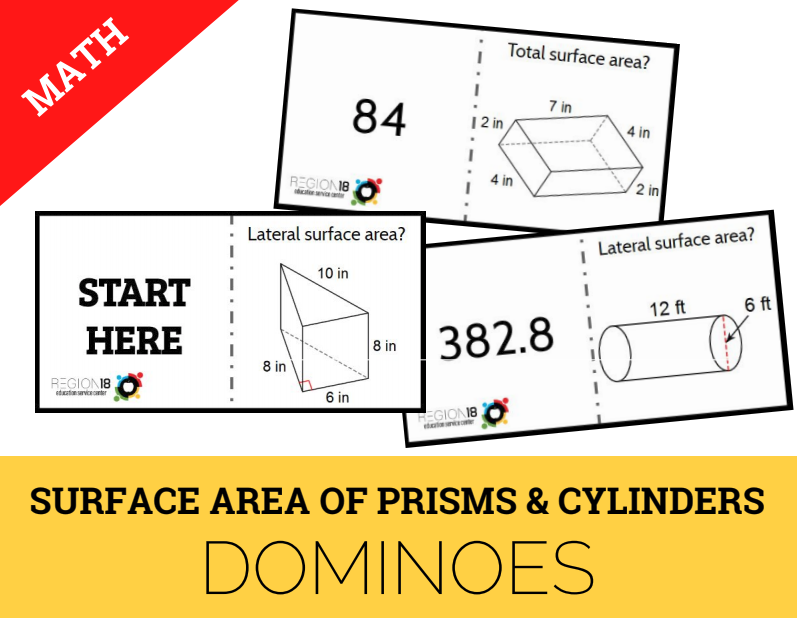Determine solutions to surface area problems involving rectangular prisms, triangular prisms, and cylinders. 8.7C Converse of Pythagorean Theorem Coloring Page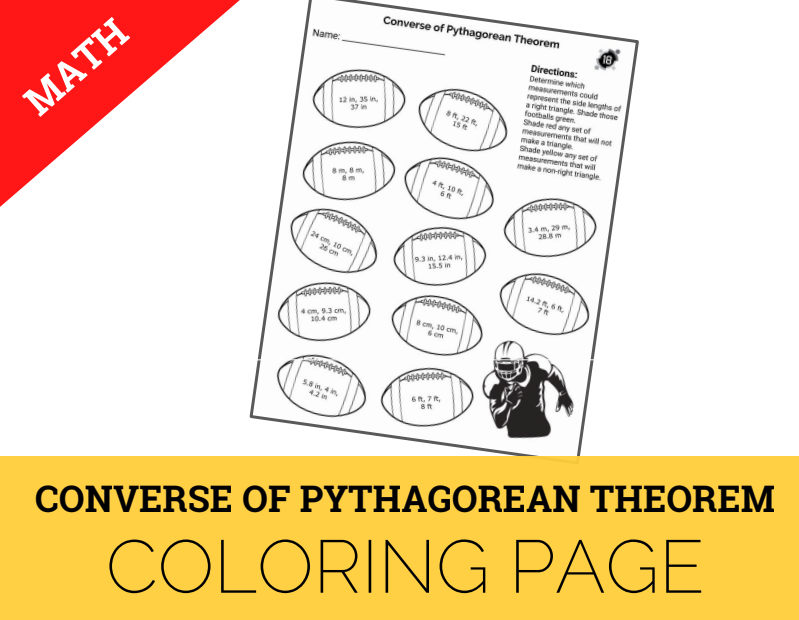Use the converse of the Pythagorean Theorem to solve problems. 8.8C Solving Equations Stacking Pyramid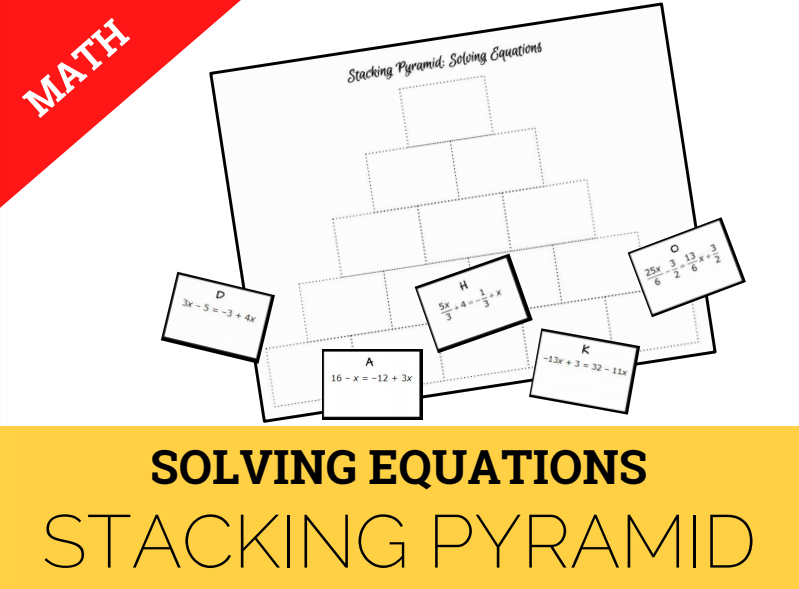Solve one-variable equations with variables on both sides of the equal sign using rational number coefficients and constants. 8.8D Angle Relationships Dominoes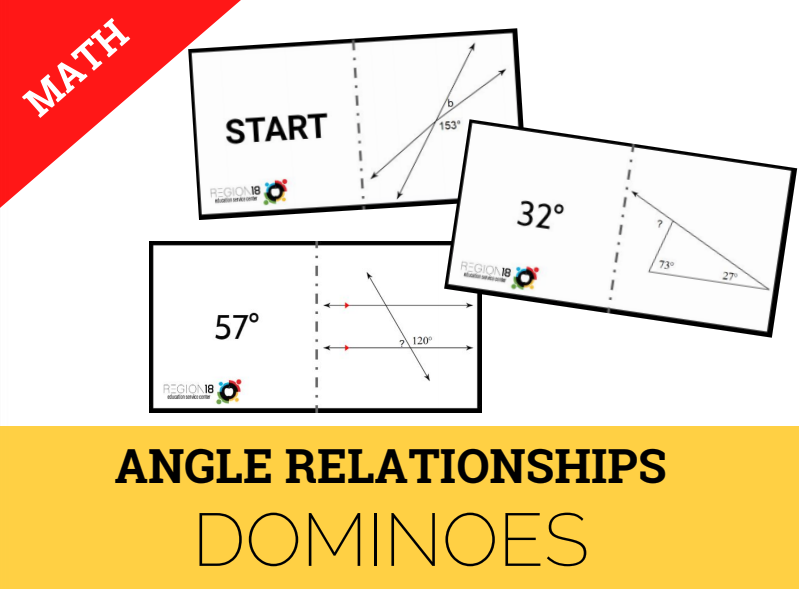Use informal arguments to establish facts about the angle sum of triangles, exterior angles of triangles, and angles created when parallel lines are cut by a transversal. 8.12D Simple & Compound Interest Task Cards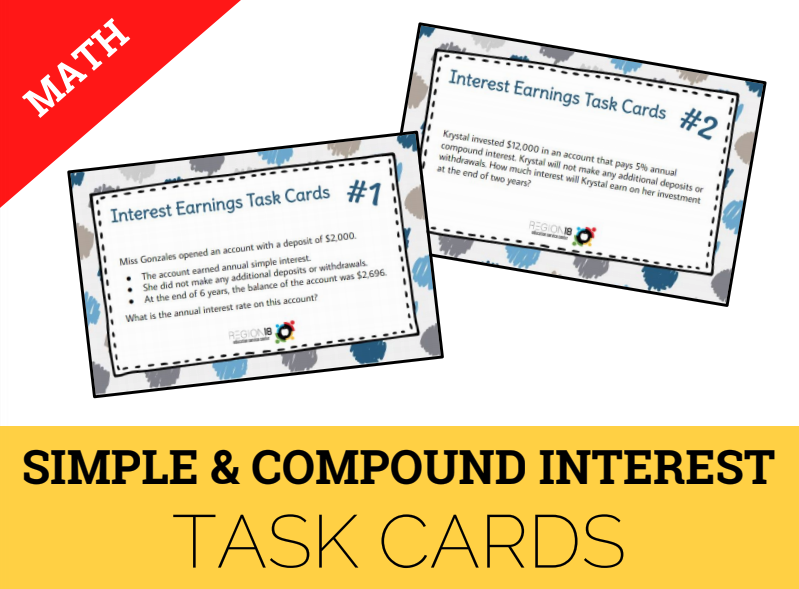Calculate and compare simple interest and compound interest earnings.